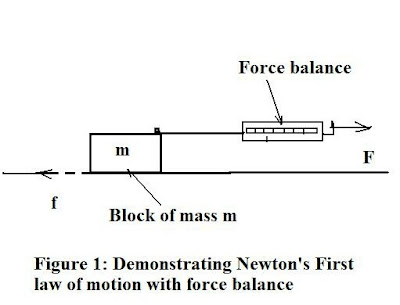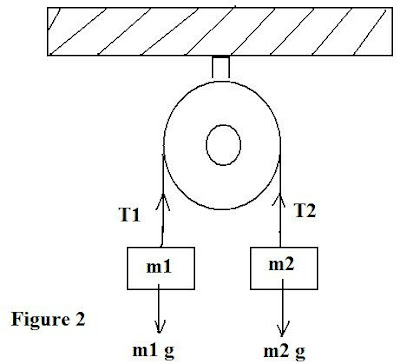## Tuesday, May 3, 2011

### Introduction to Basic Physics (Mechanics)- Pt. 1As noted in a blog two years ago, there is a strong incentive for basic physics education, especially since many physics- related issues factor into the political milieu. Thus, areas like global warming-climate change, Peak Oil and alternative energy, as well as reasons for crumbling infrastructure. In the last case, mechanics is the area of physics one needs to look at and we begin with this blog examining some fundamentals.

Mechanics can basically be reduced to Newton’s three laws of motion. Everything from a simple pulley, to a mass moving on an inclined plane, or a rocket’s flight, can be embodied by one or more of the three laws. In this chapter we recap them and also show experimental examples and some problems. Once again, the three laws of motion are:

1) Any body at rest or in uniform motion remains at rest or in uniform motion unless acted on by an outside force.

2) The acceleration of an object is directly proportional to the resulting force acting on it (F = ma)

3) If 2 bodies interact, the force that body 1 exerts on body 2 is equal and opposite to the force that body 2 exerts on body 1. (F(12) = F(21) = - F(12))

1. Demonstrations of the First Law:

To demonstrate Law (1) we use a simple block of known mass (m) to which we attach a spring-operated force balance which measures the pulling force (F) in Newtons. We then pull the mass along a level surface and record the reading on the balance. The experimental setup is shown in Fig. 1.

The critical measure is the resultant force (F – f), where f is the putative frictional force acting in the opposite direction to the applied force, F. If we find from the balance reading that (F- f) = 0, then the resultant force is zero and hence, the motion is uniform so Newton’s first law applies. The applied force F is exactly cancelled by the friction force, f. Hence, acceleration a = 0 and F = ma = 0.

A second way to demonstrate the First law experimentally is shown in the next diagram. Here we attach two equal masses, m1 and m2 around a simple pulley with a string connecting them. We will find that provided we have the setup similar to that shown in the diagram, neither m1 nor m2 move (accelerate) downward, and hence this means that the two forces: m1 g and m2 g are equal to each other, so there is no resultant force.

Another way to express this result is in terms of the string tensions, T1 and T2. In such a static case: T1 = m1 g and T2 = m2 g and since this equilibrium holds, then T1 = T2. In this case, the bodies (blocks) are at rest, and we note the 1st law states bodies “at rest or in uniform motion”. Hence, it must follow that all cases of static equilibrium are also examples of the 1st law of motion.

Next: Particular applications.